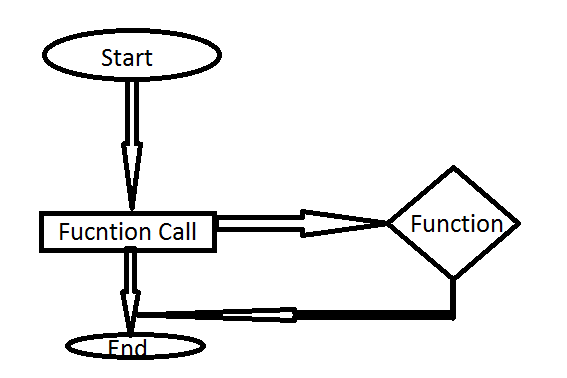# PHP FunctionsPHP Functions

A PHP function is used to execute a branch of code when needed from the pages where the function is included, and the PHP functions can call several times,

## A normal PHP function

to write a normal PHP function first declare the function then write some code inside the function or inside the scope and then call the function from anywhere of the page.

Example:

<?php
function fn_print(){
echo “Inside the php function”;
}

fn_print();
?>

** in this case it will print the string on the position from where you have called the function.

## A PHP function with passing value

Through a PHP function you can pass a single or multiple value and work with that value, a 2 variable passing and sum of them example is given below,

Example:

<?php
function fn_sum(\$a=0,\$b=0){
\$c = \$a + \$b;
echo “The sum of a and b = ” . \$c;
}

fn_sum(); // it will print The sum of a and b = 0
fn_sum(4); // it will print The sum of a and b = 4
fn_sum(2,4); // it will print The sum of a and b = 6
?>

** in this case if you are not sending any value then it will take the value which is assign on the first bresses of the function.
** and on the second case it will get the first value which is sent and the second value which is assigned on the functions first bresses
** in in case of the third example it will get the 2 both value will be taken as the sending value is the first priority.

## A PHP function with the return value

Like the above 2 functions the return type function does not just execute the code or print, it uses to return a value also which can be used after calling the function, an example is given below.

Example:

<?php
function fn_sum(\$a=0,\$b=0){
\$c = \$a + \$b;
return \$c;
}

\$e = fn_sum(2,4); //In this case the value of e will be 6

echo \$e; // It will print here 6
?>

Please try the PHP Functions by yourself and i hope that you get a clear view on php function.